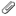#### General Information #

Author: David Plotkin
Language: ACTION!
Compiler/Interpreter: ACTION!
Published: ANALOG Issue #20

## Bounce in Action!#

Bounce, written by Joel Gluck and published in ANALOG issue 15, was a lot of fun to play with, just as Joel predicted it would be. The obvious enhancements that sprang to mind included a higher resolution screen and multiple colors. Unfortunately, higher resolution (and more than four colors) means more points to draw, and BASIC slows to a crawl. Fortunately, Action! from OSS presents an alternative, so I translated and modified the program into Action! Try punching it in; I think you'll agree that the color patterns and dynamic "ball" are fascinating to watch. To use this updated version of Bounce, you must have the Action! cartridge installed in your Atari. The program works pretty much like the original: You draw "walls" with your joystick, then hit the space bar to start the ball bouncing. Hitting the space bar again stops the bounce, so you can draw more walls with your joystick, or erase by pushing the fire button. If you press the ESCAPE key while the bouncing is stopped, you will return to the menu screen to review the commands. The program uses Graphics 11, so there are fifteen colors on the screen, and the color of the line drawn changes each time the cursor bounces. The left arrow key (CONTROL *) changes the speed of the bouncing cursor; at the highest speed it's really moving. It can go even faster if you delete the DO OD loops following the sound statements. You will lose the sounds of the bounce if you do, however. So have fun with this juiced-up version of Bounce.
Action! Listing
```MODULE

; BOUNCE from ANALOG magazine
; Issue #15
; in GTIA Mode 11

BYTE key=764,x,y,console=53279,
attract=77
CARD ctr
INT A,B

PROC wallchex()

IF x>78 THEN x=78 FI
IF y>190 THEN y=190 FI
IF x<1 THEN x=1 FI
IF y<1 THEN y=1 FI

RETURN

PrintE("BOUNCE from Analog Issue #15")
PrintE("       in GTIA mode 11")
PrintF("%E* Use stick to draw walls,%E")
PrintF("* Hold trigger to erase,%E")
PrintF("* Hit ESC to clear screen,%E")
PrintE("* Hit SPACE to bounce.")
PrintE("* ARROWS  control ball speed")
Print("Press any key to continue.")
key=255
While key=255 DO OD
key=255

RETURN

PROC drawscreen()

BYTE curs=752
Graphics (0)
curs=1
Graphics(11)
curs=1
SetColor(4,0,4) ;SetColor(4,0,0)
Color=15
Plot(0,0)
DrawTo(79,0)
DrawTo(79,191)
Drawto(0,191)
DrawTo(0,0)

RETURN

PROC flash()

color=9
Plot(x,y)
FOR ctr=0 to 300 DO OD
color=0
Plot(x,y)
FOR ctr=0 to 300 DO OD

RETURN

PROC bounce()

BYTE fate=53770,L=,PA,PB,G,
kolor=,time=

color=9
A=1
B=1
Plot(x,y)
DO
IF key=33 THEN key=255 RETURN FI
WHILE Locate(x+A,y+B)<15
DO
color=kolor
Plot(x,y)
x==+A
y==+B
wallchex()
color=9
Plot(x,y)
L==+1
FOR ctr=0 to 5*time DO OD
OD
IF key=7 THEN
key=255
time==-32
FI
Sound(0,L*4+20,10,8)
PA=Locate(x+A,y)
PB=Locate(x,y+B)
FOR ctr=0 to 100 DO OD
SndRst()
L=0
IF PA>2 AND PB>2 THEN
A=-A
B=-B
ELSEIF PA>2 AND PB<3 THEN
A=-A
color=2
Plot(x,y)
y=y+B
color=9
Plot(x,y)
ELSEIF PB>2 AND PA<3 THEN
B=-B
color=2
Plot(x,y)
x=x+A
color=9
Plot (x,y)
ELSEIF fate>127 THEN
B=-B
ELSE A=-A
FI
kolor==+1
IF kolor>14 THEN
kolor=1
FI
attract=0
OD
RETURN

PROC draw()

BYTE qq

drawscreen()
x=40
y=95
DO
IF key=28 THEN
key=255
drawscreen()
ELSEIF key=33 THEN
key=255
bounce()
FI
IF Stick(0)=15 THEN
flash()
ELSEIF Stick(0)=7 THEN
x=x+1
ELSEIF Stick(0)=6 THEN
x=x+1
y=y-1
ELSEIF Stick(0)=14 THEN
y=y-1
ELSEIF Stick(0)=5 THEN
x=x+1
y=y+1
ELSEIF Stick(0)=11 THEN
x=x-1
ELSEIF Stick(0)=10 THEN
x=x-1
y=y-1
ELSEIF Stick(0)=13 THEN
y=y+1
ELSEIF Stick(0)=9 THEN
x=x-1
y=y+1
FI
wallchex()
IF Strig(0)=0 THEN
color=0
flash()
ELSE
color=15
FI
Plot(x,y)
IF Stick(0)<>15 THEN
qq=Strig(0)
Sound(0,(200-x-y)*qq,8+2*qq,4)
FOR ctr=0 to 1000 DO OD
SndRst()
FI
OD
RETURN
```

bounce in ACTION/bounceinaction.PDFbounce in ACTION/bounceinaction.djvuOnly authorized users are allowed to upload new attachments.

### List of attachments

Kind Attachment Name Size Version Date Modified Author Change note
pdf
bounceinaction.PDF 137.7 kB 1 20-Feb-2010 22:06 Carsten Strotmann
djvu
bounceinaction.djvu 89.1 kB 1 20-Feb-2010 22:06 Carsten Strotmann
gif
bounceinaction.gif 43.3 kB 1 20-Feb-2010 22:06 Carsten Strotmann
png
bounceinaction.png 89.9 kB 1 20-Feb-2010 22:07 Carsten Strotmann
« This page (revision-6) was last changed on 03-Feb-2023 16:21 by Gromit
G’day (anonymous guest) My Prefs
Articles
Hardware
Software
Applications
Memory Map Topic list
MicroUSB
AllNews
FAQ
Index
Languages
Code

What's New?

Voice and Data Cassettes
Atari Program Exchange (APX)
Atari Learning System Software
PLATO (Programmed Logic for Automated Teaching Operation)
Podcasts
Official country specific Atari software

Thanks
Rarity 10
Statistics
All content in the Wiki is licensed under Creative Commons Share Alike License, unless otherwise noted.